Shipra Saxena — March 31, 2021

## Objective

• Naive Bayes is a fast, easy to understand, and highly scalable algorithm.
• Understand the working of Naive Bayes, its types, and use cases.

## Introduction

Naive Bayes is one the most popular and beginner-friendly algorithms that anyone can use. In this article, we are going to explore the Naive Bayes Algorithm.

Note: If you are more interested in learning concepts in an Audio-Visual format, We have this entire article explained in the video below. If not, you may continue reading.

## Concept  Behind Naive Bayes

Let’s First understand how Naive Bayes works through an example. We have a dataset with some features Outlook, Temp, Humidity, and Windy, and the target here is to predict whether a person or team will play tennis or not. So, we are representing features as X like X1, X2, and so on. Similarly, the classes are represented as C1 and C2.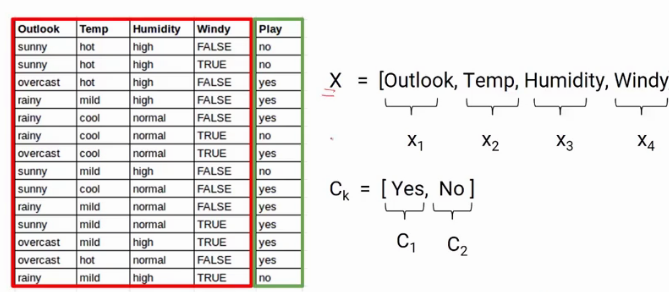In Naive Bayes for every observation, we determine the probability that it belongs to class 1 or class 2. For example, here we first find out the probability that the person will play given that Outlook is Sunny, Temperature is Hot, Humidity is High and it is not windy as shown below. Later, we will also calculate the probability that the person will not play given the same conditions. This is repeated for all the rows.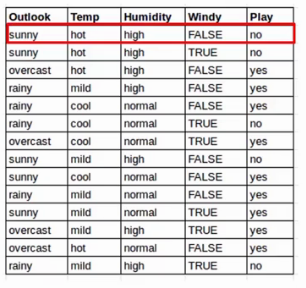So this is in a way calculating the conditional probability, where we try to predict the class based on the conditions or the features here.

### Conditional Probability

Recall the formula of conditional probability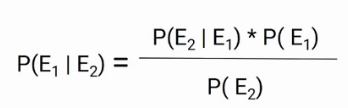In this case, we have the probability of E1 for a given condition E2. Here, we are predicting the probability of class1 and class2 based on the given condition. If I try to write the same formula in terms of classes and features, we will get the following equation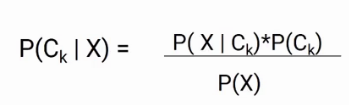Now we have two classes and four features, so if we write this formula for class C1, it will be something like this.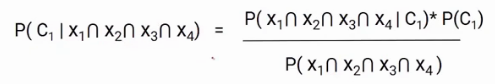Here, we replaced Ck with C1 and X with the intersection of X1, X2, X3, X4. You might have a question, why we are taking the intersection? It’s because we are taking the situation when all these features are present at the same time.

The Naive Bayes algorithm assumes that all the features are independent of each other or in other words all the features are unrelated. With that assumption, we can further simplify the above formula and write it in this form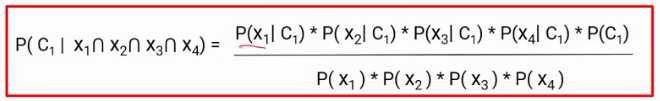This is the final equation of the Naive Bayes and we have to calculate the probability of both C1 and C2.

For this particular example-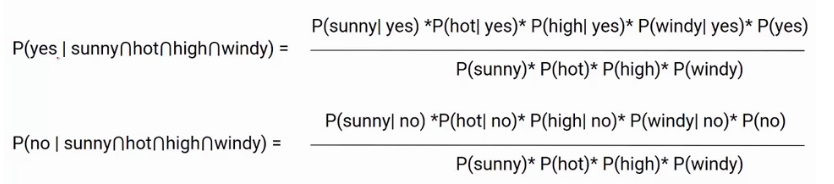This means we have to find the probability of a person will play or not based on the given features. Whichever the probability is higher is taken as the final class.

## Types of Naive Bayes

Now let’s discuss different types of Naive Bayes algorithm and which is used when. So, we have three types

### Gaussian Naive Bayes

This type of Naive Bayes is used when variables are continuous in nature. It assumes that all the variables have a normal distribution. So if you have some variables which do not have this property, you might want to transform them to the features having distribution normal.

### Multinomial Naive Bayes

Next comes the multinomial Naive Bayes. This is used when the features represent the frequency.

Suppose you have a text document and you extract all the unique words and create multiple features where each feature represents the count of the word in the document. In such a case, we have a frequency as a feature. In such a scenario, we use multinomial Naive Bayes.

It ignores the non-occurrence of the features. So, if you have frequency 0 then the probability of occurrence of that feature will be 0 hence multinomial naive Bayes ignores that feature. It is known to work well with text classification problems.

### Bernoulli Naive Bayes

This is used when features are binary. So, instead of using the frequency of the word, if you have discrete features in 1s and 0s that represent the presence or absence of a feature. In that case, the features will be binary and we will use Bernoulli Naive Bayes.

Also, this method will penalize the non-occurrence of a feature, unlike multinomial Naive Bayes.

• Here are some advantages of the Naive Bayes algorithm.
• This algorithm is easier to build and simpler to understand.
• It is much faster than the other algorithms as it is just calculating the probabilities.
• Naive Bayes is easily scalable hence widely used in the industry.
• It is a popular choice for text classification problems.

### Points to be Remembered

There are some important points you must keep in mind while implementing the Naive Bayes algorithm.

All the features are considered independent or unrelated to each other. So this algorithm will not calculate the interaction among the features that might be a drawback.

Another important thing is when you use Gaussian naive Bayes, the algorithm assumes that all the continuous features have the normal distribution.

At last, you should remove all the highly correlated features from the dataset otherwise they will be counted twice and increase the processing.

## Applications of Naive Bayes

If we talk about some of the applications of the Naive Bayes algorithm.

It is majorly used in real-world apps that require responding to the user’s requests instantaneously. Other common applications that you would come across would be filtering spams from the mails, document classification, or sentiment prediction

## End Notes

To summarize, in this article we saw the working of the Naive Bayes algorithm along with its types. We also saw the advantages and use cases of the algorithm.

If you are looking to kick start your Data Science Journey and want every topic under one roof, your search stops here. Check out Analytics Vidhya’s Certified AI & ML BlackBelt Plus Program

If you have any questions let me know in the comment section!